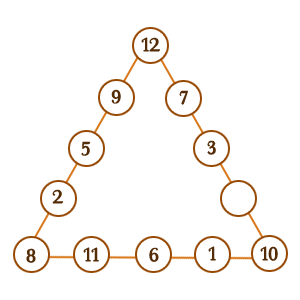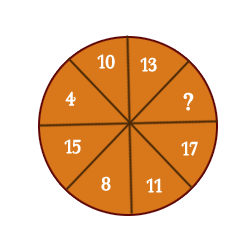## Numbers Puzzles

3. Place the numbers 1 - 12 in the twelve circles below so the sum of each side of the triangle is 36. I will give you a head start by placing some of the numbers for you. (The numbers may be used once only).Answer
 4 .
4. What is the missing number in the pie below?Answer
 The missing number is 6.The two numbers opposite each other always total 21 : 15 + 6 = 21.